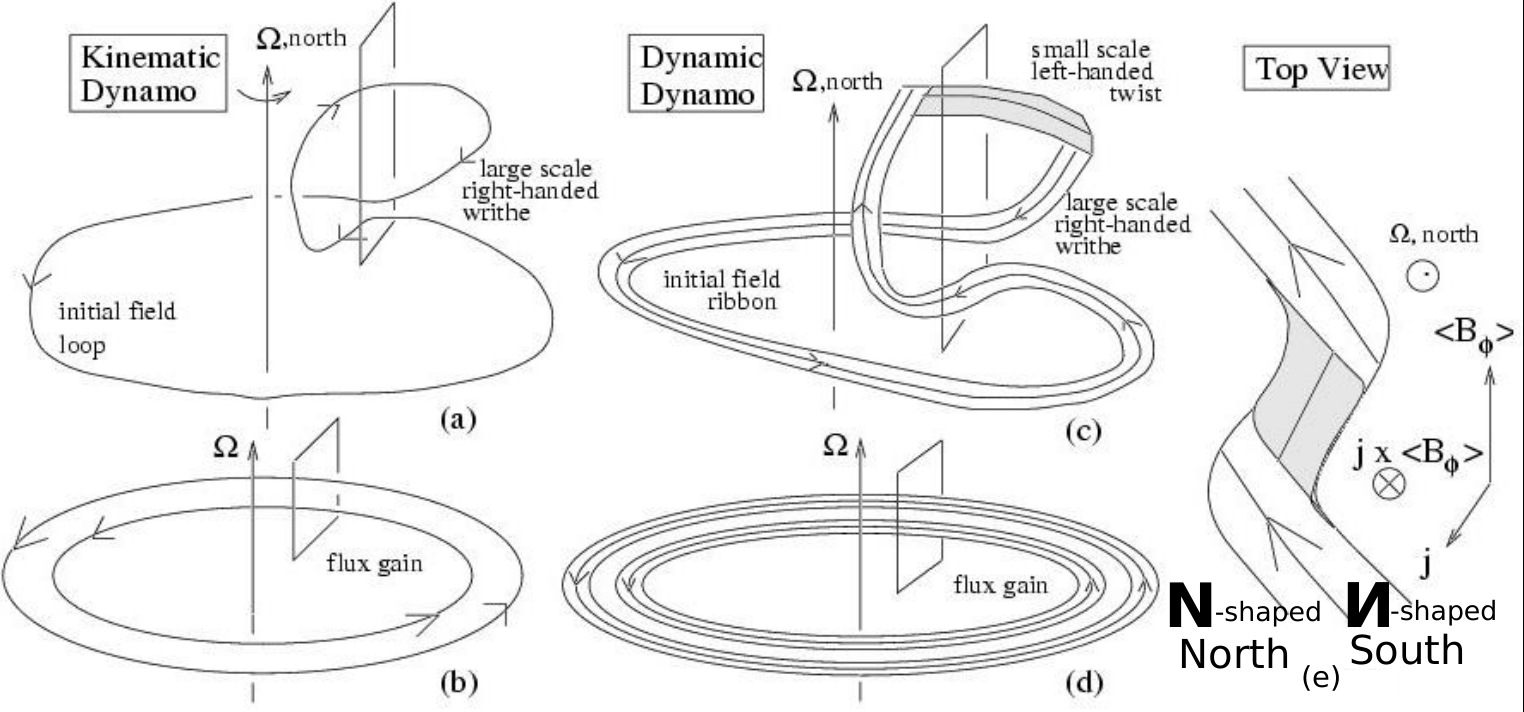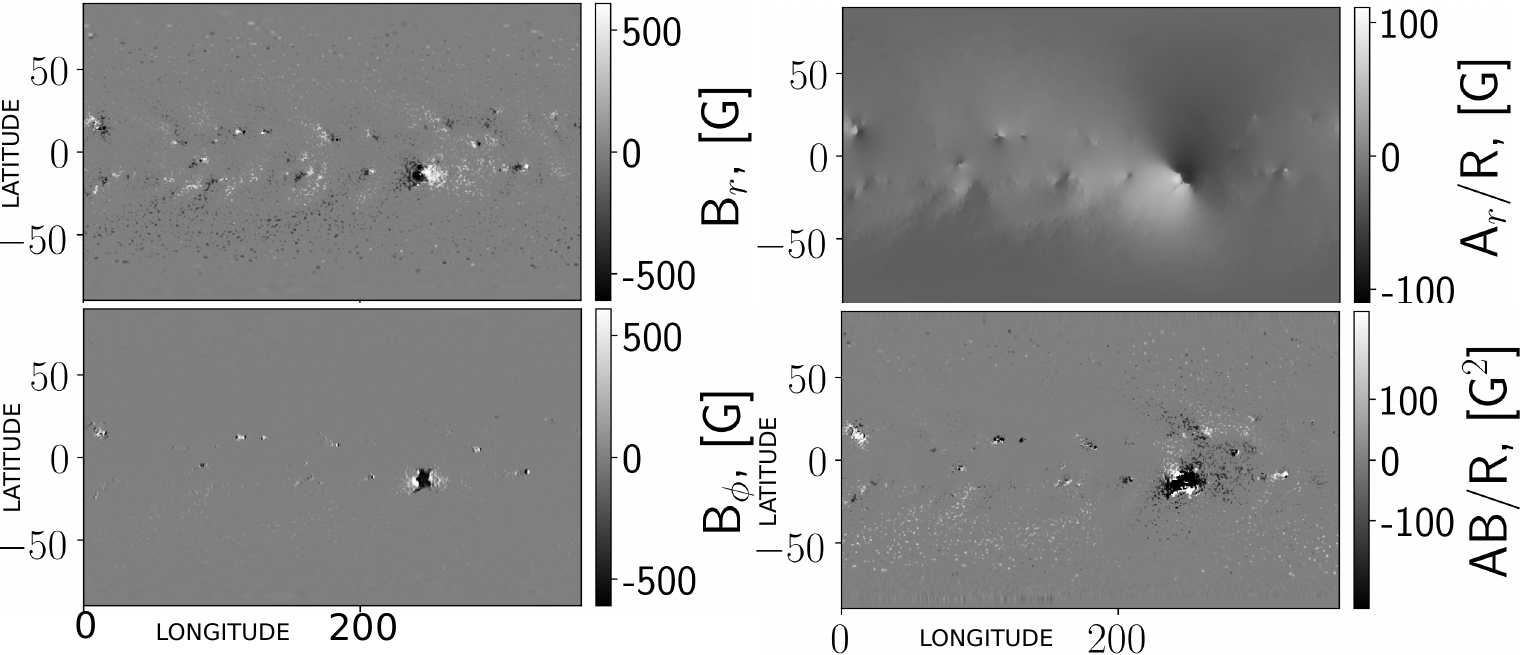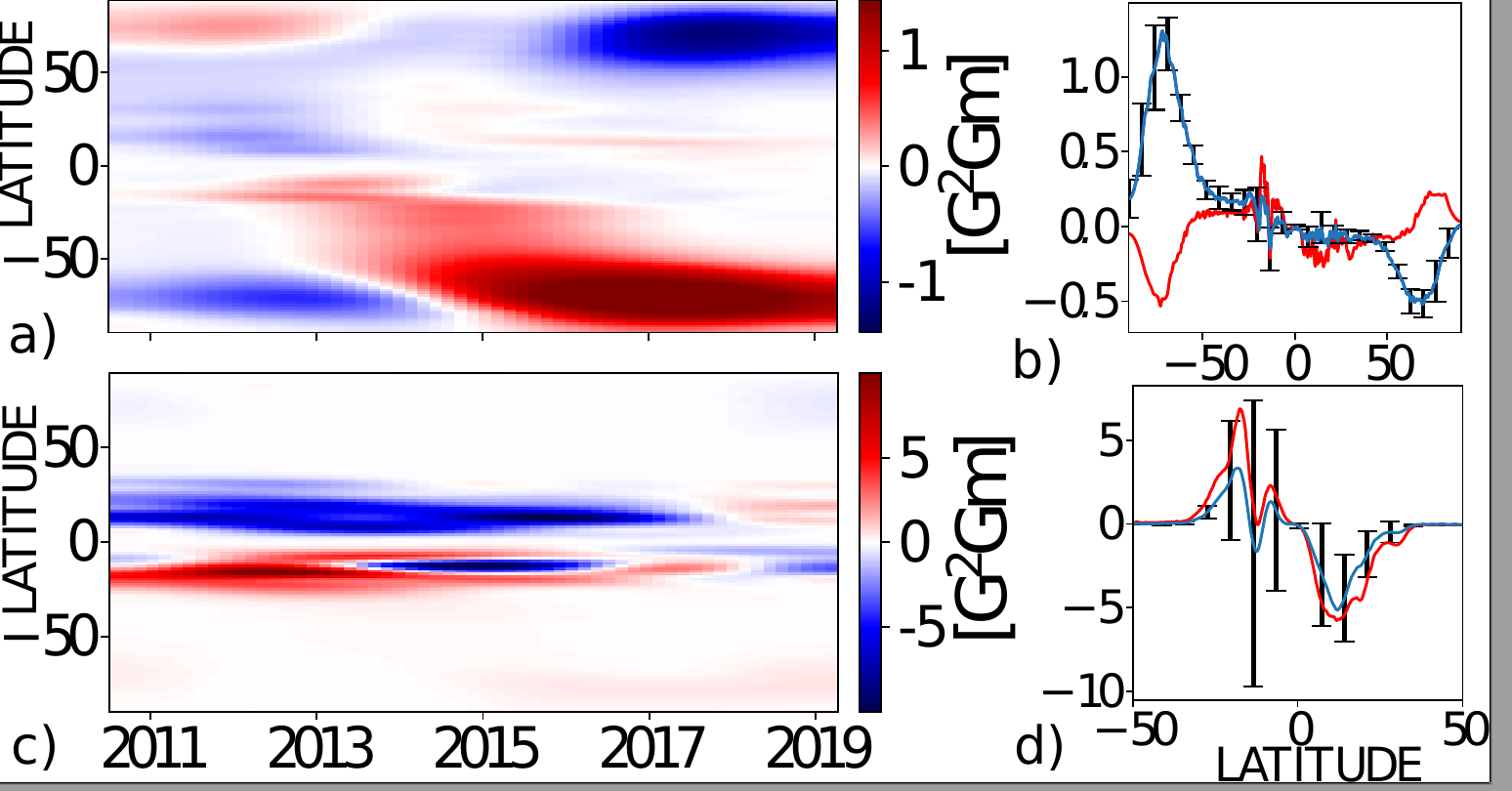# 128. Evolution of Magnetic Helicity in Solar Cycle 24

Contributed by V.V. Pipin. Posted on June 25, 2019

V.V. Pipin1, A.A. Pevtsov2, Yang Liu3 and A.G. Kosovichev4

1 Institute of Solar-Terrestrial Physics, Russian Academy of Sciences, Irkutsk, 664033, Russia
2 National Solar Observatory 3665 Discovery Drive, 3rd Floor Boulder, CO 80303, USA
3 Stanford University, Stanford, CA, USA
4 Department of Physics, New Jersey Institute of Technology, Newark, NJ 07102, USA

Magnetic helicity conservation is believed to be rather significant for the nonlinear stage of the large-scale dynamo in solar-type stars. Figure 1 is a sketch of the general idea of how the magnetic helicity interplays with the dynamo processes in the stellar convection zone. It illustrates two basic theoretical ideas about the magnetic helicity in the solar dynamo. First, it shows the hemispheric magnetic helicity rule; and second, it shows that helicity of the global and local magnetic field of the Sun should have, in general, opposite signs.Figure 1| Significance of magnetic helicity for solar-type dynamos1. Panels a and b show the linear stages of the dynamo process. Following the sketch of the “rope” dynamo2, it includes two basic steps: (a) writhe a loop of the toroidal magnetic field into a poloidal loop, and (b) the poloidal loop is stretched and folded into azimuthal plane. Panels (c) and (d) show how the magnetic helicity conservation affects the stage (a) because the global writhe is accompanied by the local twist of the global magnetic field in the flux-tube cross-section plane, and the direction of twist is opposite to the writhe. Directions of writhe and twist, which are shown in panel (e), have opposite signs in the northern and southern hemispheres of the Sun.

Magnetic helicity is an integral measure of topological properties of the magnetic field in a closed volume$\mathrm{V}$,$H_{M}=\int\mathbf{A}\cdot\mathbf{B}d\mathrm{V}$, where$\mathbf{A}$ is the magnetic vector potential,$\mathbf{B}=\boldsymbol{\nabla}\times\mathbf{A}$, and$\mathbf{B}$ is confined to the volume$\mathrm{V}$. Therefore, in general, to study magnetic helicity, we need the volume magnetic field distributions. The$\mathbf{A}\cdot\mathbf{B}$ is the magnetic helicity density. The spatially or temporarily averaged$\overline{\mathbf{A}\cdot\mathbf{B}}$ characterizes the mean magnetic helicity or the mean linkage of the magnetic field in the domain of averaging3. This quantity perfectly suits for comparison of the observational data with results of theoretical dynamo models.

In Ref , to determine the$\overline{\mathbf{A}\cdot\mathbf{B}}$ we use synoptic maps of the vector magnetic field obtained by the Helioseismic and Magnetic Imager (HMI) on board Solar Dynamics Observatory (SDO). We decompose the magnetic field and its vector-potential into the mean and fluctuating parts:$\mathbf{B}=\mathbf{\overline{B}}+\mathbf{b}$,$\mathbf{A}=\mathbf{\overline{A}}+\mathbf{a}$, where the small letters represent the small-scale fluctuations and the capital ones with over-bars are the large-scale fields. In the case of solar magnetism, the time scale of mean magnetic field corresponds to the solar cycle period and small-scale magnetic field varies on much shorter intervals corresponding to the typical time scales of solar active regions. The solar dynamo predominantly operates in an axisymmetric dynamo regime. Therefore, we can express the relationship between the magnetic helicity density of the large- and small-scale magnetic fields as follows:$\mathbf{\overline{a\cdot\mathbf{b}}}=\overline{\mathbf{A}\cdot\mathbf{B}}-\mathbf{\overline{A}\cdot\mathbf{\overline{B}}}.$ Thus, the small-scale magnetic helicity density$\mathbf{\overline{a\cdot\mathbf{b}}}$, includes magnetic fields from all range of scales except the axisymmetric magnetic field.

To determine the magnetic helicity density, we employ a decomposition of the vector magnetic field into toroidal and poloidal components using scalar potentials$S$ and$T$ :$\mathbf{B} =\boldsymbol{\nabla}\times\left(\hat{\mathbf{r}}T\right)+\boldsymbol{\nabla}\times\boldsymbol{\nabla}\times\left(\hat{\mathbf{r}}S\right)$$= -\frac{\hat{r}}{r}\Delta_{\Omega}S+\hat{\theta}\left(\frac{1}{\sin\theta}\frac{\partial T}{\partial\phi}-\frac{\sin\theta}{r}\frac{\partial F_{S}}{\partial\mu}\right)+\hat{\phi}\left(\sin\theta\frac{\partial T}{\partial\mu}+\frac{1}{r\sin\theta}\frac{\partial F_{S}}{\partial\phi}\right)$

where$\Delta_{\Omega}=\frac{\partial}{\partial\mu}\sin^{2}\theta\frac{\partial}{\partial\mu}+\frac{1}{\sin^{2}\theta}\frac{\partial^{2}}{\partial\phi^{2}}$,$\mu=\cos\theta$ and$\theta$ is the polar angle, and$F_{S}=\partial\left(rS\right)/\partial r$. After finding$S$,$T$ and$F_{S}$， we can determine components of vector potential$\mathbf{A}$. Calculations were done in the spectral space using the spherical harmonic decomposition. Figure 2 illustrates reconstruction of vector magnetic potential for the Carrington rotation CR2156. The large active region in the southern hemisphere produced the negative magnetic helicity density. It is opposite to the hemispheric helicity rule. Nearby the ARs of the same helicity sign occupy the big portion of solar surface on both sides of the solar equator.Figure 2| Synoptic maps of the vector magnetic field components$B_{r,\phi}$ from SDO/HMI for CR 2156, the reconstructed potential$A_{r}$, and the magnetic helicity density.

Figure 3 shows the time-latitude diagrams of the magnetic helicity density of the large-scale field,$\bar{\mathbf{A}}\cdot\bar{\mathbf{B}}$ and the small-scale non-axisymmetric magnetic field$\mathbf{\overline{a\cdot\mathbf{b}}}$. Both the large- and small-scale magnetic fields of the Sun show the hemispheric helicity rule. Generally, evolution of$\mathbf{\overline{a\cdot\mathbf{b}}}$ in Cycle 24 agrees with results of Ref  for the current helicity of solar active regions in cycle 23. In our data we see that the magnetic helicity density of the large- and small-scale fields often show the same sign in each hemisphere. This may be due to the asymmetric development about the equator in Solar Cycle 24. This results in the equatorial parity breaking in the large-scale magnetic field and vector potential components. Please see a more detailed discussion in the paper4.Figure 3| Magnetic helicity in Solar Cycle 24: (a) The time-latitude evolution of the large-scale magnetic helicity density,$\mathbf{\overline{A}\cdot\mathbf{\overline{B}}}$; (b) the mean latitudinal profile of the large-scale magnetic helicity density with 95% confidence interval for the standard error, the red line shows the average profile for the first half of the cycle including CR2097-2156; (c) shows the same as (a) for the azimuthal averaging of the small-scale magnetic helicity density$\mathbf{\overline{a\cdot\mathbf{b}}}$; (d) shows the same as (b) for the$\mathbf{\overline{a\cdot\mathbf{b}}}$.

The hemispheric helicity rule and bi-helical distributions of the solar magnetic fields are essential properties of the solar dynamo operating in the convection zone. We find that in Solar Cycle 24 these properties of solar dynamo show a complicated evolution in the way which is not expected in any current dynamo models. The suggested method of the magnetic helicity reconstruction can be applied to other stars where the low-degree modes of the vector magnetic field distributions can be used to calculate the magnetic vector potential and magnetic helicity density of the large-scale stellar magnetic field.

References:
 Blackman, E. G., & Brandenburg, A. 2003, ApJL, 584, L99
 Childress, S., & Gilbert, A. G. 1995, Stretch, twist, fold: the fast dynamo (Springer)
 Arnol’d, V. I., & Khesin, B. A. 1992, Annual Review of Fluid Mechanics, 24, 145
 Pipin, V. V., Pevtsov, A. A., Liu, Y., & Kosovichev, A. G. 2019, ApJ, 877, L36
 Zhang, H., Sakurai, T., Pevtsov, A., Gao, Y., Xu, H., Sokoloff, D. D., & Kuzanyan, K. 2010, MNRAS, 402, L30